# SPM Mathematics 2016, Paper 2 (Questions 13 & 14)

Question 13 (12 marks):
Diagram 13.1 shows three triangles RPQ, UST and RVQ, drawn on a Cartesian plane.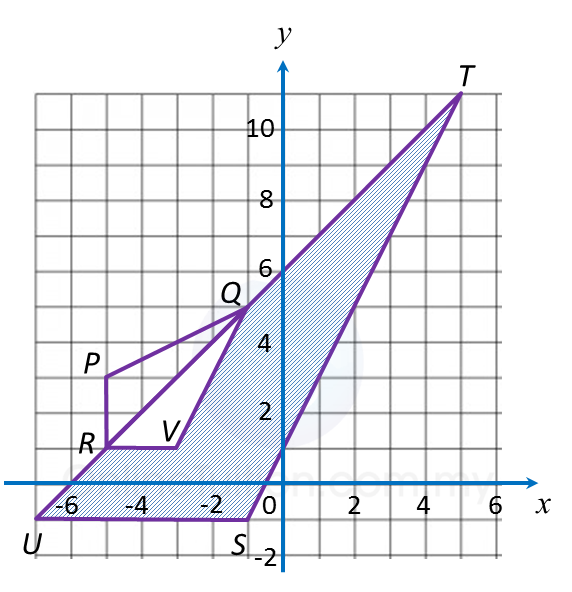Diagram 13.1

(a) Transformation R is a rotation of 90o, clockwise about the centre O.
Transformation T is a translation $\left(\begin{array}{l}2\\ \text{3}\end{array}\right)$ .
State the coordinates of the image of point B under each of the following transformations:
(i) Translation T2,
(ii) Combined transformation TR.

(b)
(i) Triangle UST is the image of triangle RPQ under the combined transformation VW.
Describe in full the transformation:
(a) W   (b) V

(ii) It is given that quadrilateral RPQ represents a region of area 15 m2.
Calculate the area, in m2, of the region represented by the shaded region.

Solution: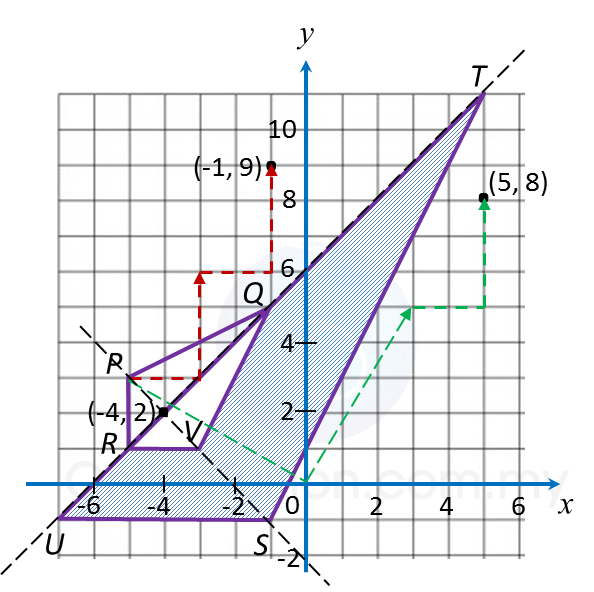(a)
(i) (–5, 3) → T → (–3, 6) ) → T → (–1, 9)
(ii) (–5, 3) → R → (3, 5) → T → (5, 8)

(b)(i)(a)
W: A reflection in the line URQT.

(b)(i)(b)

(b)(ii)
Area of UST = (Scale factor)2 x Area of RPQ
= 32 x area of RPQ
= 32 x 15
= 135 m2

Therefore,
= Area of UST – area of RPQ
= 135 – 15
= 120 m2

Question 14 (12 marks):
Diagram 14 shows a histogram which represents the mass, in kg, for a group of 100 students.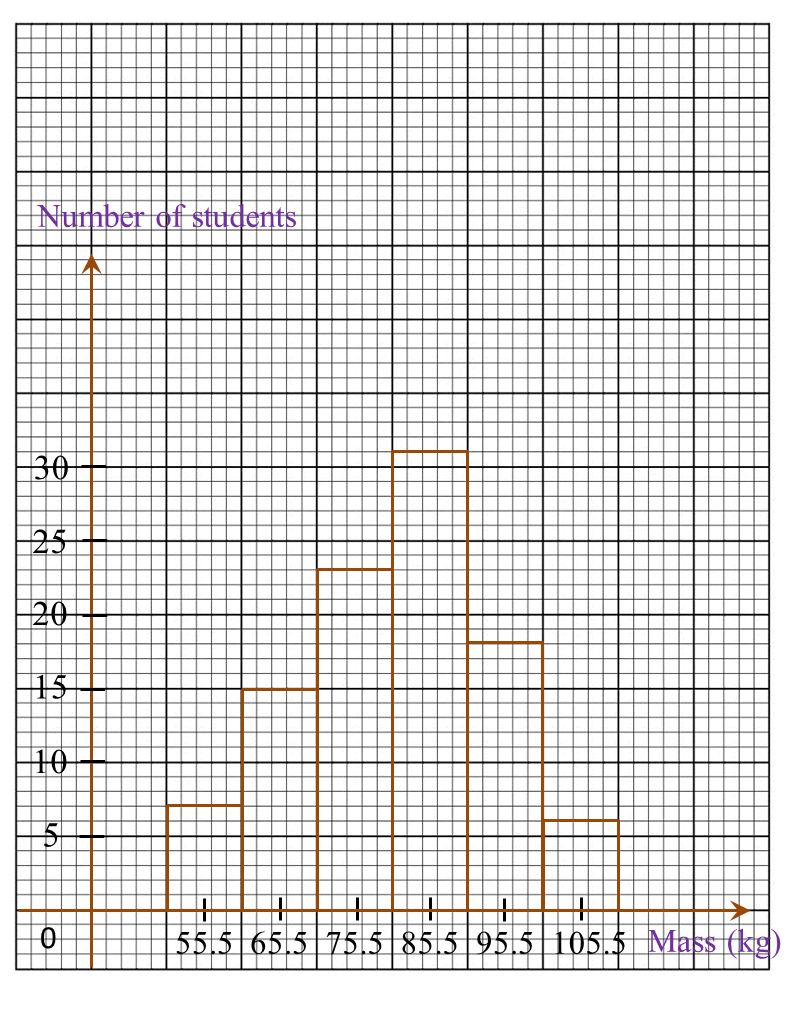Diagram 14

(a)
Based on Diagram 14, complete Table 14 in the answer space.

(b)
Calculate the estimated mean mass of a student.

(c)
For this part of the question, use graph paper. You may use a flexible curve ruler.
Using a scale of 2 cm to 10 kg on the horizontal axis and 2 cm to 10 students on the vertical axis, draw an ogive for the data.

(d)
Based on the ogive drawn in 14(c), state the third quartile.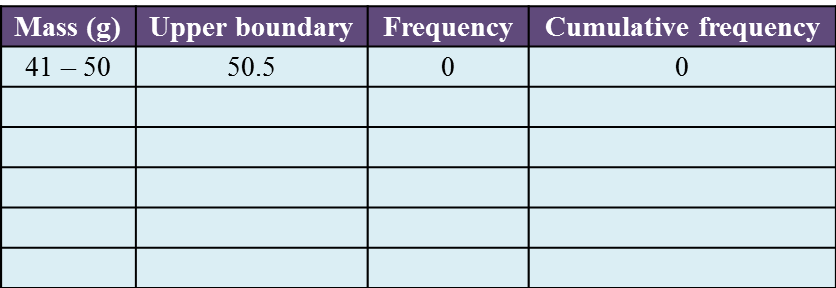Solution:
(a)(b)(c)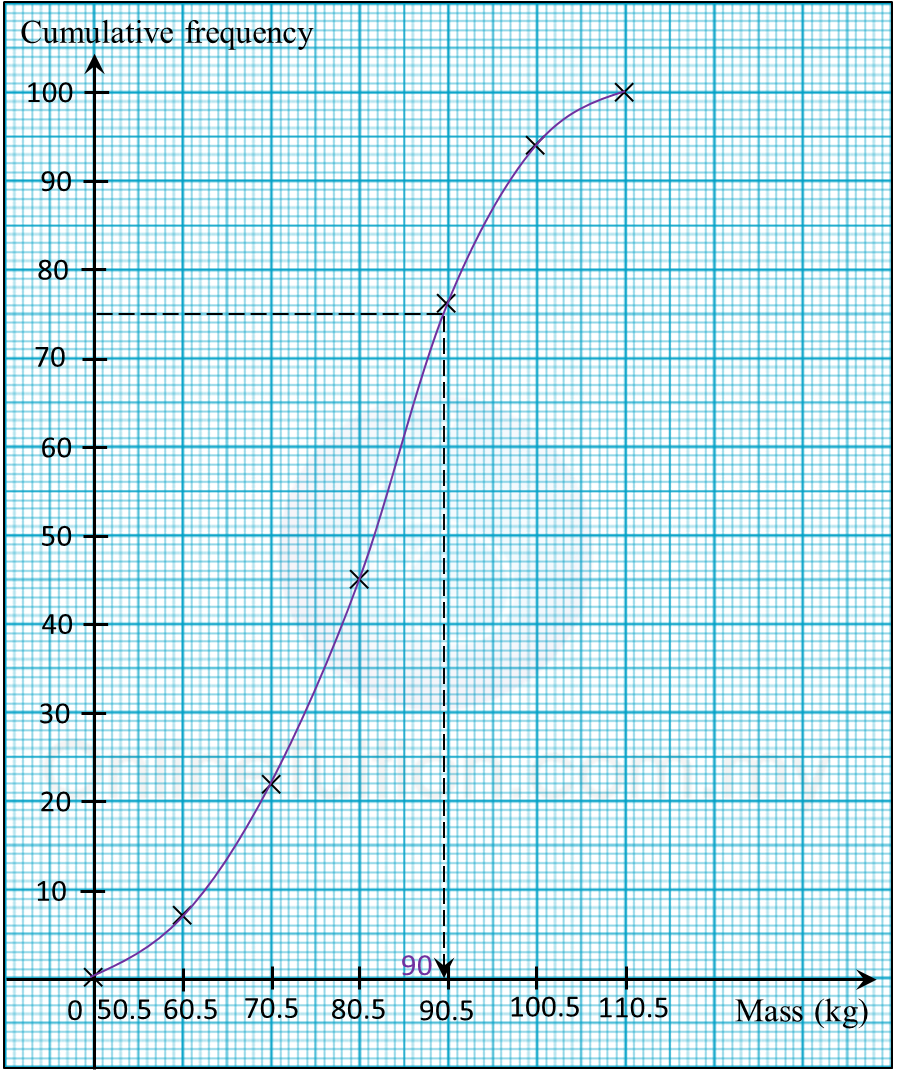(d)
Third quartile
= 75th student
= 90.0 kg